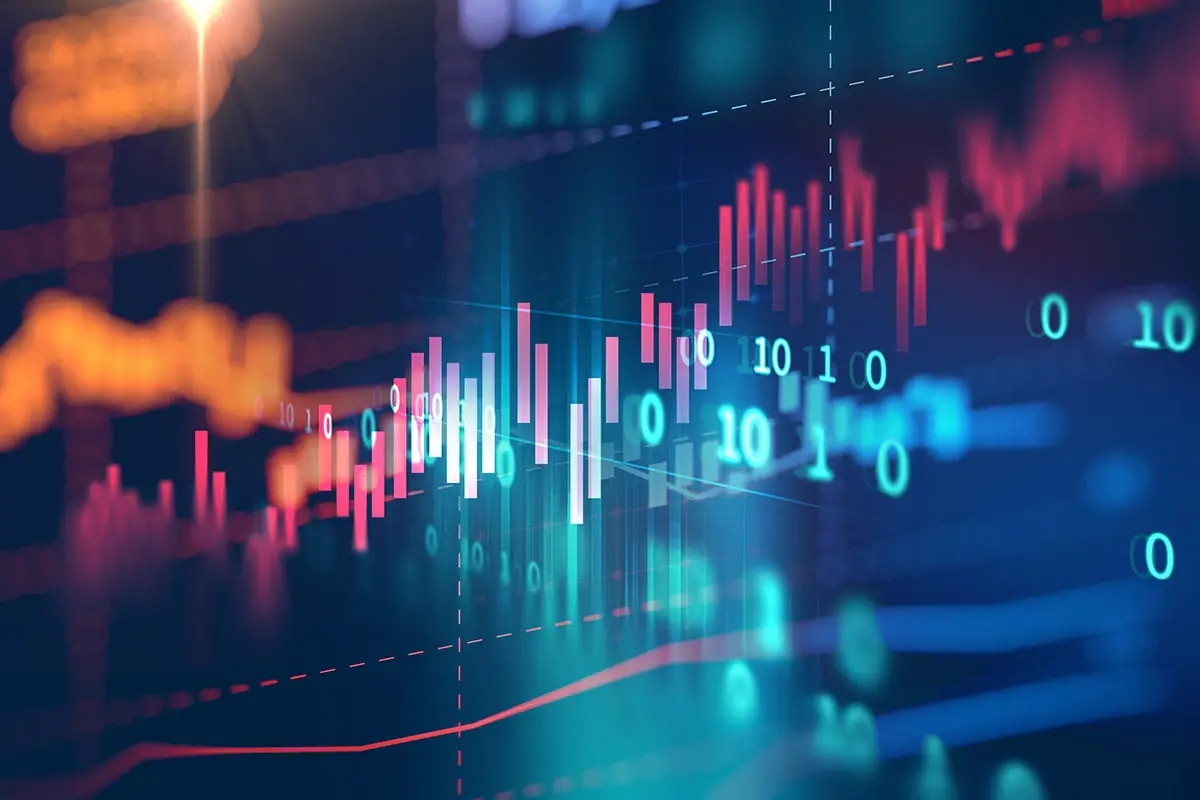# Analysis of Sequential Data

The course will cover various techniques for analyzing time series data, starting with visualizations, then moving on to methods for identifying trends and seasonal patterns. It will also delve into more advanced statistical techniques like exponential smoothing and ARIMA. The course will include practical examples using real-world data sets and will teach students how to extract useful features from digital signals for classification. The course will have a hands-on approach, with students using Python programming to apply the concepts they have learned in case studies. The course will focus on a probabilistic approach and will also include labs to help students gain hands-on experience with statistical models like HMMs and DNNs for recognizing and classifying time series.

## Objectives

By the end of the course, students will be able to:

• Visualize time series data and identify key features
• Evaluate the accuracy of forecasts
• Model trends, seasonal patterns, and non-stationarity using exponential smoothing and ARIMA models
• Perform estimation, selection, and probabilistic prediction with these models
• Analyze digital signals in different domains, such as time and spectral
• Use modern deep learning techniques to classify digital signals.

## Lectures

• Lesson 1: Getting started with Python + libraries
• Lesson 2: Fundamentals in statistics, probability and stochastic processes
• Lesson 3: Correlations and related functions
• Lesson 4: Decomposition of Time Series
• Lesson 5: ARMA, ARIMA models and their use in modeling
• Lesson 6: Smoothing
• Lesson 7:Time series models of heteroscedasticity (ARCH, GARCH, financial models)
• Lesson 8: Introduction to spectral analysis
• Lesson 9: Estimating the spectrum
• Lesson 10: Hidden Markov Models (HMMs)
• Lesson 11: State Space Models: Kalman
• Lesson 12: Deep learning approaches: for timeseries: RNNs, LSTM

## Labs

• Lab 1: Basic statistics with Python
• Lab 2: Correlations
• Lab 3: Decomposition of Time Series
• Lab 4: ARMA, ARIMA
• Lab 5: Smoothing, forecasting and estimation
• Lab 6: GARCH
• Lab 7: Spectral analysis
• Lab 8: Estimating the spectrum
• Lab 9: HMM
• Lab 10: Recurrent Neural Networks (Vanilla and LSTM)

• Homework 1:

## Software

• Python release 3.11 (December 2022)
• Anaconda Navigator (Jupyter, Ipython, Spyder,…)
• Libraries: Numpy, Pandas, Matplotlib, Scikit-learn, Scipy

## Exam and notation

• Kind of exam: written
• Duration of the exam: 120 minutes
• Permissible aids: 2 handwritten summary sheets + scientific calculator
• Final grade: 80% exam, 20% project(s)

## Language of the course

• Instruction is given in English
• Documentation is available in English
• The examination is available in English

## Textbooks

Time Series Analysis and Its Applications
Shumway, Robert H ; Stoffer, David S
Springer International Publishing, 2017

Forecasting: Principles and Practice, online
Rob J Hyndman and George Athanasopoulos

Analysis of Financial Time Series, online
Ruey S. Tsay
John Wiley & Sons, Inc.

Spoken language processing guide to algorithms and system development by Xuedong Huang, Alex Acero, Hsiao-Wuen Hon, Amazon

Introduction to Time Series and Forecasting
Peter J. Brockwell Richard A. Davis
Springer